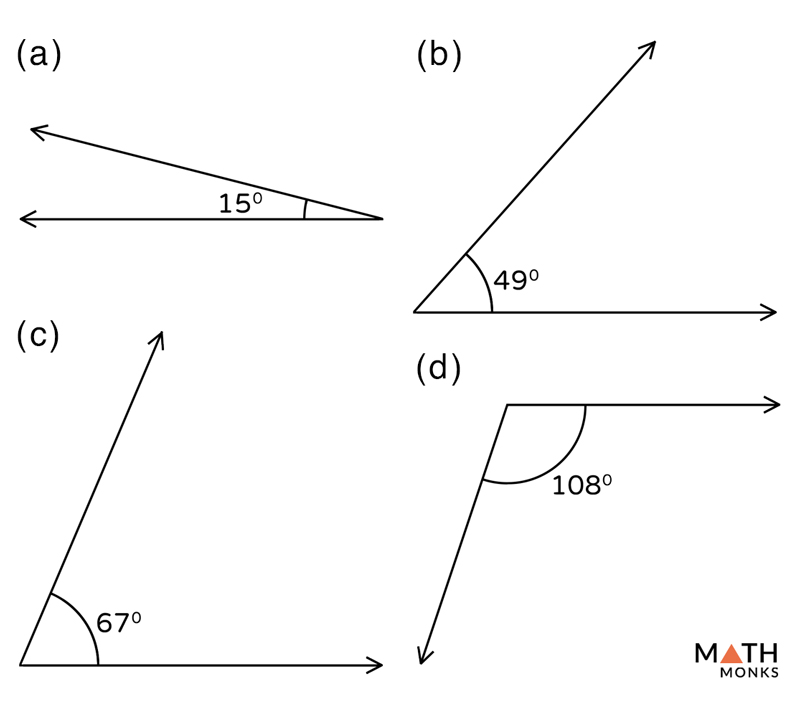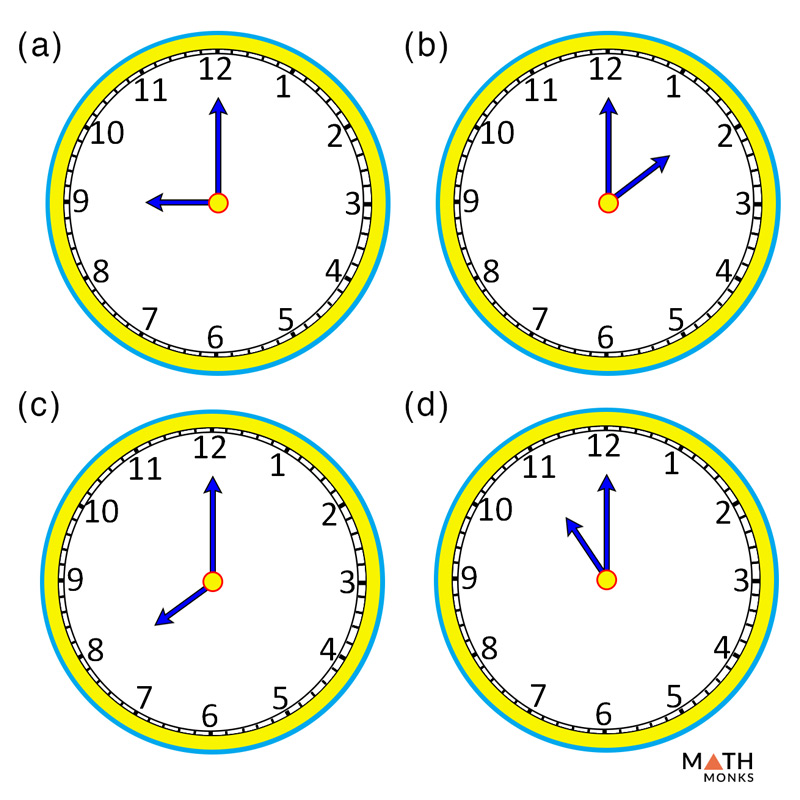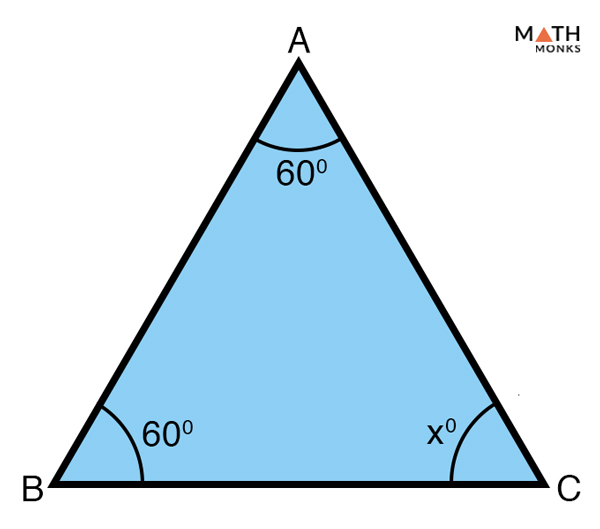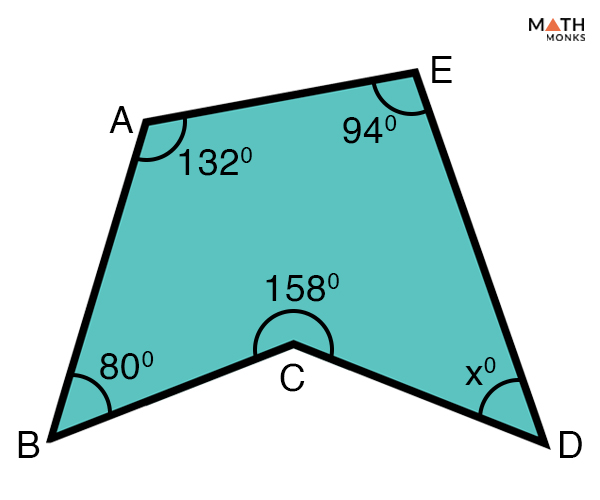# Acute Angle

## What is an Acute Angle

An acute angle is defined as an angle that measures more than 0° and less than 90°. In other words, any angle smaller than a right angle is an acute angle.

Two or more acute angles can form a right angle (equals 90°) or an obtuse angle (greater than 90°). When the two acute angles make a right angle each measuring 45°, they are called congruent acute angles.

A few more examples of acute angle are shown below:

## Acute Angles in Real Life

• All angles in an acute angle triangle, two angles each in obtuse, and right angle triangle
• Alphabets A, K, M, N, V, W, X, Y, and Z
• Objects of everyday use such as funnel, coat hanger, hands of wall clock showing 10’ o clock, a partly-open cupboard door, and kitchen tongs
• Study materials such as a pencil point, opened book, divider, compass, and set squares
• Geometric shapes such as arrowhead, ‘<‘, and ‘>’ signs
• A slice of watermelon
• Branches of a tree
• Spokes of a bicycle
• The opened mouth of a crocodile
• The opened beak of a bird
• The intersection of a crossroad showing ‘one way’ or ‘no right turn’Choose from the given options the acute angles.

Solution:

As we know,
Acute angles are angles less than 90°,
Hence, options (a), (b), and (c) are acute angles.Which of the following options given in the clock timing gives an acute angle?

Solution:

As we know,
Acute angles are angles less than 90°,
Hence, options (b) and (d) are acute angles.Find x in the given triangle and state whether it is an acute angle triangle

Solution:

As we know,
The sum of the interior angles in a triangle is 180°,
Thus,
In △ABC,
∠ABC + ∠BCA + ∠CAB = 180°, here ∠ABC = 60°, ∠CAB = 60°
60° + ∠BCA + 60° = 180°
∠BCA = 180° – (60° + 60°)
∠BCA = (x°) = 60°
Since all three angles ∠ABC, ∠BCA, ∠CAB measure less than 90°, △ABC is an acute angle triangleIdentify the acute angles in the given polygon ABCDE.

Solution:

As we know,
Sum of the interior angles of a pentagon = 540°
Now,
∠ABC +∠BCD + ∠CDE + ∠DEA +∠EAB = 540°
80° + 158° + ∠CDE + 94° + 132° = 540°
∠CDE = 540° – 464°
∠CDE = (x°) = 76°
Thus, ∠ABC and ∠CDE measure less than 90° and are acute angles.

## FAQs

Q1. How many acute angles are found in an acute triangle?

Ans. There are three acute angles in an acute triangle.

Q2. How many acute angles does a right triangle have?

Ans. There are two acute angles in a right triangle.

Q3. How many acute angles are in an obtuse triangle?

Ans. There are two acute angles in an obtuse triangle.

Q4. How many acute angles are in a rhombus?

Ans. Two among the four angles in a rhombus are acute.

1.Alma B says: